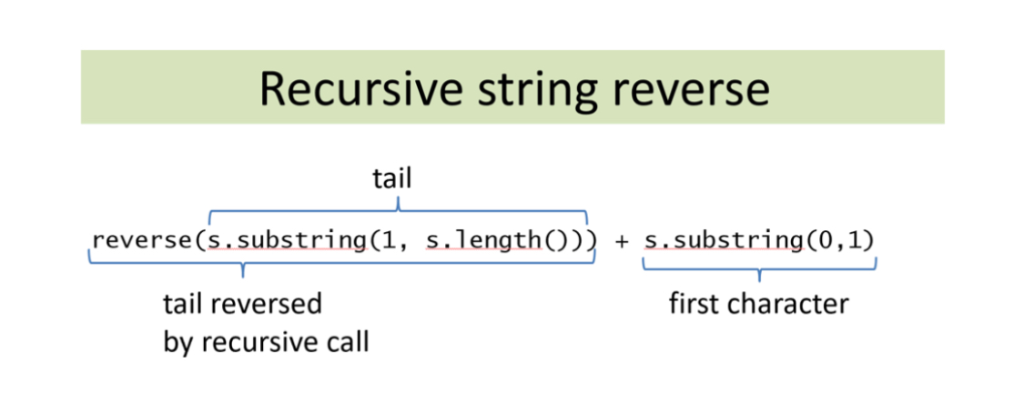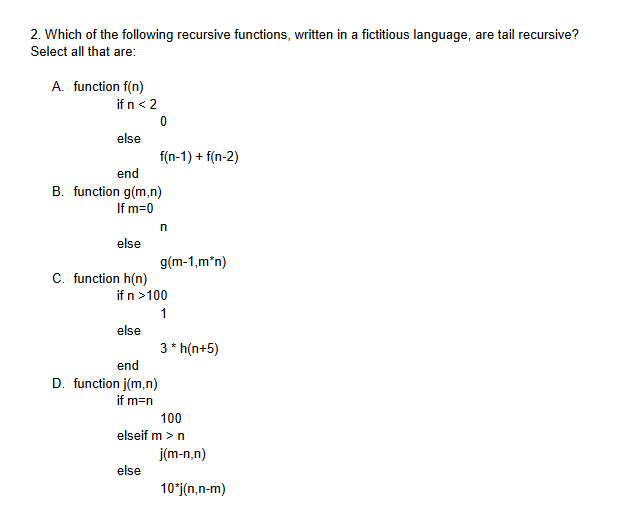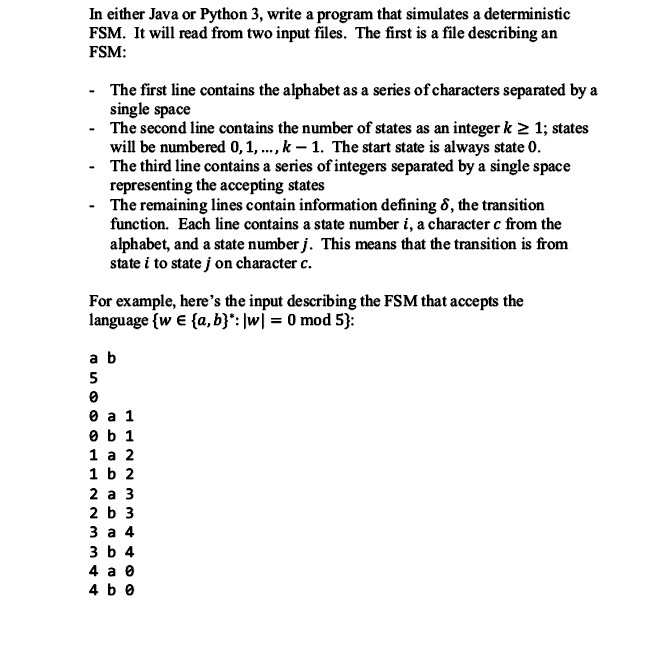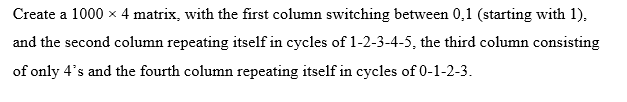# Recursive string reverse tail reverse(s.substring (1, s.length())ג + s.substring(0,1) first chara...

Please create a functioning Java program which includes the following:Recursive string reverse tail reverse(s.substring (1, s.length())ג + s.substring(0,1) first character tail reversed by recursive call

```//Main.java
public class Main {
public static String reverse(String s){
if(s==null || s.length() == 0){
return "";
}
else{
return reverse(s.substring(1,s.length()))+s.substring(0,1);
}
}

public static void main(String[] args) {
System.out.println(reverse("hello"));
}
}
```olleh##### Add Answer of: Recursive string reverse tail reverse(s.substring (1, s.length())ג + s.substring(0,1) first chara...
Similar Homework Help Questions
• ### Recursive String Methods

Recursive String MethodsIn this problem, you will write several static methods that use recursion to operate on a String.Requirements:The methods that you write must be purely recursive. The use of iteration (i.e., for, while, or do-while loops) is not allowed.The only built-in String methods that you may use are charAt, length, equals, and substring. No use of other String methods is allowed. In addition, make sure tofollow any additional restrictions specified in the problem.Do not use any global variables --...

• ### java recursive function

public class StringNode {private char ch;private StringNode next;/*** Constructor*/public StringNode(char c, StringNode n) {ch = c;next = n;}/*** convert - converts a standard Java String object to a linked-list* string and returns a reference to the linked-list string*/public static StringNode convert(String s) {if (s.length() == 0)return null;StringNode firstNode = new StringNode(s.charAt(0), null);StringNode prevNode = firstNode;StringNode nextNode;for (int i = 1; i < s.length(); i++) {nextNode = new StringNode(s.charAt(i), null);prevNode.next = nextNode;prevNode = nextNode;}return firstNode;}public static void printEveryOther(StringNode str)This method should...

• ### Qhuestion 6 i.Write a recursive function reverseConcatenate that takes as input a list with string entries and returns one long string which is the concatenation of all the string entries in reverse o...

Qhuestion 6 i.Write a recursive function reverseConcatenate that takes as input a list with string entries and returns one long string which is the concatenation of all the string entries in reverse order. For instance, reverseConcatenate([’how’, ’are’, ’you?’]) should return ’you?arehow’.

• ### 2. Which of the following recursive functions, written in a fictitious language, are tail recursive? Select all that are A. function f(n) ifn<2 else f(n-1) + f(n-2) end If m=0 else B. function g(m...2. Which of the following recursive functions, written in a fictitious language, are tail recursive? Select all that are A. function f(n) ifn<2 else f(n-1) + f(n-2) end If m=0 else B. function g(m,n) g(m-1,m'n) C. function h(n) if n 100 else 3 h(n+5) end D. function j(m.n) IT m=n 100 j(m-n,n) 10 j(n,n-m) elseif mn else 2. Which of the following recursive functions, written in a fictitious language, are tail recursive? Select all that are A. function f(n) ifn

• ### Build a server application which will reverse the order of a string received from a client. When client connects, a new thread is started to handle an interactive dialog in which the client sends in a...

Build a server application which will reverse the order of a string received from a client. When client connects, a new thread is started to handle an interactive dialog in which the client sends in a string input by the user and the server thread sends back the letters of the string in reverse order. Allow the client to send 10 Strings. In Java.

• ### You will implement and test several short recursive methods below. With the proper use of recursi...this can be done in one class or two separate classes,in java thanks! You will implement and test several short recursive methods below. With the proper use of recursion, none of these methods should require more than a dozen lines of code. Test all these in the same class. Keep adding methods and testing until all are working 5. A Fractal Pattern Examine this pattern of stars and blanks, and write a recursive method that can generate patterns such as...

• ### In either Java or Python 3, write a program that simulates a deterministic FSM. It will read from two input files. The first is a file describing an FSM The first line contains the alphabet as a seri...In either Java or Python 3, write a program that simulates a deterministic FSM. It will read from two input files. The first is a file describing an FSM The first line contains the alphabet as a series of characters separated by a single space - The second line contains the number of states as an integer k 2 1; states will be numbered 0,1,..., k -1. The start state is always state O The third line contains a series...

• ### In either Java or Python 3, write a program that simulates a deterministic FSM. It will read from two input files. The first is a file describing an FSM The first line contains the alphabet as a seri...In either Java or Python 3, write a program that simulates a deterministic FSM. It will read from two input files. The first is a file describing an FSM The first line contains the alphabet as a series of characters separated by a single space - The second line contains the number of states as an integer k 2 1; states will be numbered 0,1,..., k -1. The start state is always state O The third line contains a series...

• ### Create a 1000 x 4 matrix, with the first column switching between 0,1 (starting with 1), and the ...Solve using Matlab. Provide actual code. Create a 1000 x 4 matrix, with the first column switching between 0,1 (starting with 1), and the second column repeating itself in cycles of 1-2-3-4-5, the third column consisting of only 4's and the fourth column repeating itself in cycles of 0-1-2-3 Create a 1000 x 4 matrix, with the first column switching between 0,1 (starting with 1), and the second column repeating itself in cycles of 1-2-3-4-5, the third column consisting of...

• ### JAVA String Program Problem #4 Assistance Neededx.øi5ave a JAVA string Project that I can not get to work.Below are the Requirements, Example to Solve the Program Project and the JAVA Code:Requirements:In this project, you will work with Java String. You are to write a program that does the following tasks:1) Ask the user for the name of the input file and the name of the output file2) Read a string from the input file (assume that the string is there)3)Determine its reverse string and write both...

Need Online Homework Help?